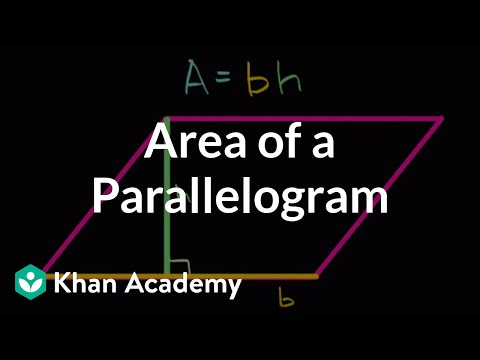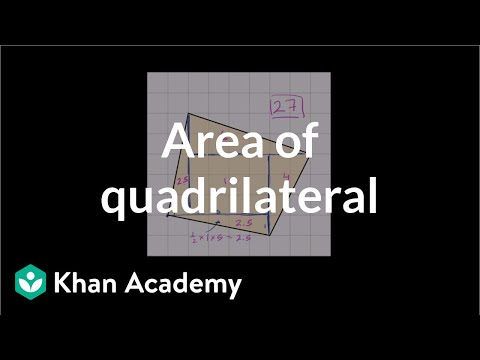Video

# Area of a triangle (Full video)

Description: Understand why the formula for the area of a triangle is one half base times height, which is half of the area of a parallelogram. In another video, we saw that, if we're looking at the area of a parallelogram, and we also know the length of a base, and we know its height, then the area is still going to be base times height. We said, "Hey, let's take this "little section right over here." So we took that little section right over there, and then we move it over to the right-hand side, and just like that, you see that, as long as the base and the height is the same, as this rectangle here, I'm able to construct the same rectangle by moving that area over, and that's why the area of this parallelogram is base times height.

### Other videos you might be interested in### Area of a parallelogram (Full video)